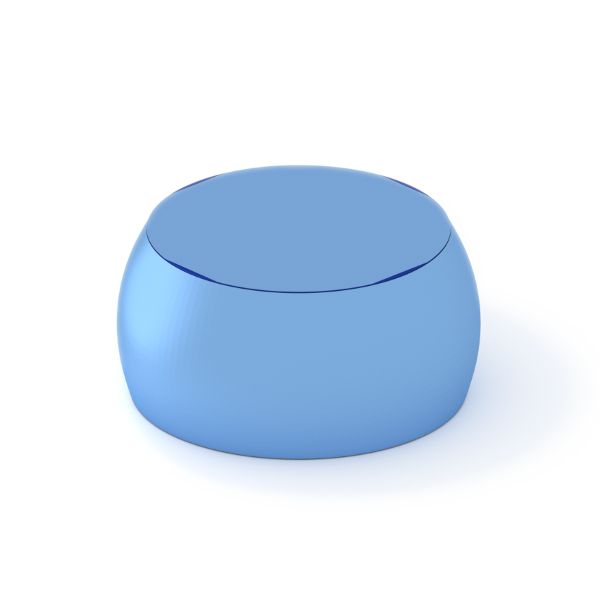### Sphere

A full sphere.#### Constructor

Sphere(R)


Parameters:

#### Usage

As for any other Form factor.

#### Implementation

Class Sphere inherits from the interface class IFormFactor .

Form factor is computed as

$$F(\mathbf{q})=4\pi R^3 \exp(iq_z R) \dfrac{\sin(qR) - qR \cos(qR)}{(qR)^3} ,$$ with the notation $$q := \sqrt{q_x^2 + q_y^2+ q_z^2}.$$

Volume has been validated against $$V=\dfrac{4\pi}{3} R^3.$$

More general:

#### Example

Scattering by uncorrelated spheres for horizontal incidence:Generated by Examples/ff/Sphere.py , Examples/fq/Sphere.py .

#### History

This form factor is well known; it goes back to Lord Rayleigh. In IsGISAXS, it has been implemented as form factor “Full sphere” [manual, Eq. 2.35; Renaud 2009, Eq. 226]. In BornAgain, named “FullSphere” up to version 1.19.